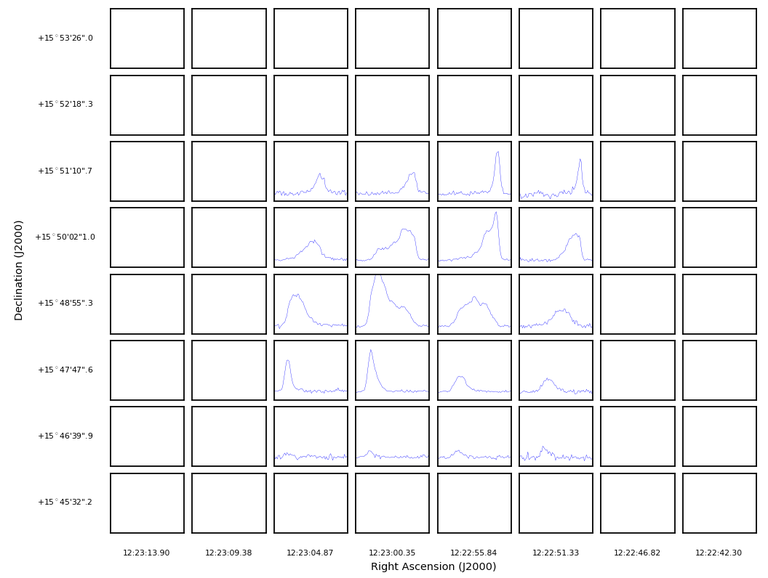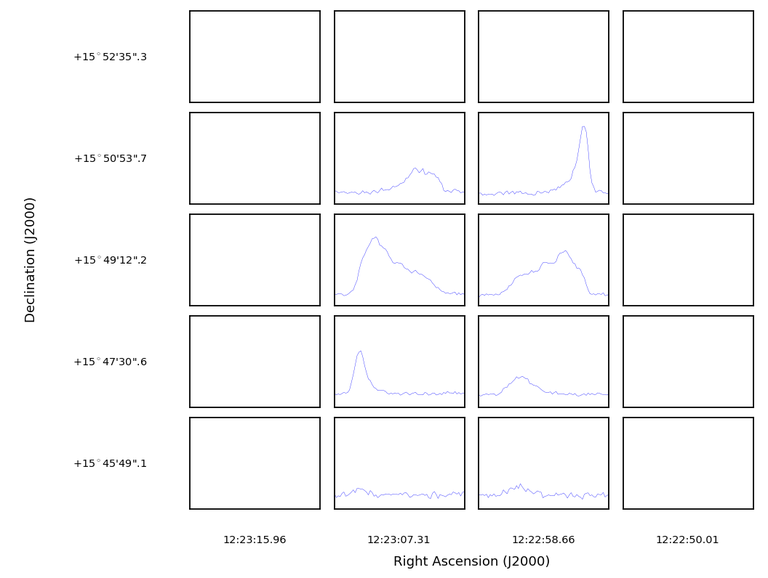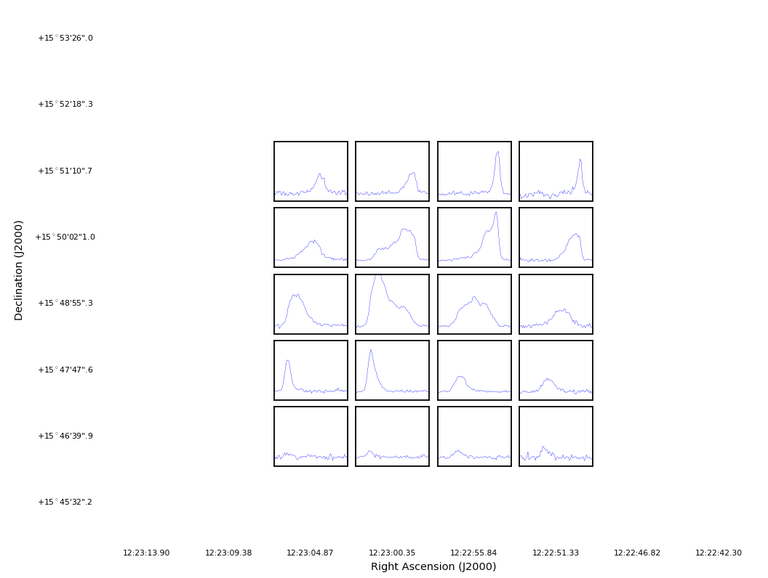# plotprofilemap

plotprofilemap(imagename, figfile='', overwrite=False, transparent=False, pol=0, spectralaxis='', restfreq='', plotrange='', title='', linecolor='b', linestyle='-', linewidth=0.2, separatepanel=True, plotmasked='empty', maskedcolor='gray', showaxislabel=False, showtick=False, showticklabel=False, figsize='', numpanels='')[source]

Makes profile map

[Description] [Examples] [Development] [Details]

Parameters
• imagename (path) - Input image name (CASA image or FITS)

• figfile (string=’’) - Output figure name

figfile != ''
• overwrite (bool=False) - Overwrite existing figfile

• transparent (bool=False) - Output transparent figure

• pol (int=0) - Polarization component to be plotted

• spectralaxis (string=’’) - Type of spectral axis

spectralaxis = velocity
• restfreq (string=’’) - Rest frequency

• plotrange (string=’’) - Spectral axis range to plot

• title (string=’’) - Title of the plot

• linecolor (string=’b’) - Line color

• linestyle (string=’-’) - Line style

• linewidth (double=0.2) - Line width in points

• separatepanel (bool=True) - Separate plots

• showaxislabel (bool=False) - Show axis labels on the bottom left panel

• showtick (bool=False) - Show axis ticks

showtick = True
• showticklabel (bool=False) - Show axis tick labels on the bottom left panel

• figsize (string=’’) - Size of the figure

• numpanels (string=’’) - Number of panels

Description

Warning

There are Known Issues for plotprofilemap.

The plotprofilemap makes a spectral profile map from a specified image. The task accepts both a CASA image or a FITS cube as an input. Output is to the screen, or to image file (specified with figfile).

It is necessary to specify existing CASA image or FITS cube as an imagename. Otherwise, the task will fail. If figfile is specified, the profile map is saved as an PNG image. Please set overwrite to False if you don’t want to overwrite existing file.

Selection of data is enabled through polarisation only (since this task operates in image data, not measurement set format).

The plot parameters can be manipulated via plotrange, title, separatepanel, showaxislabel, showtick, figsize; the line style can be specified through linecolor, linestyle, linewidth, and the actual number of sub-plots is specificed with numpanels.

The number of panels along the horizontal and vertical direction can be specified via the parameter numpanels. It should be a string containing numerical values indicating the number of panels. If only one number is given it will be applied to both axes. If you want to provide different numbers to horizontal and vertical axes, you should give two numbers as a string separated by comma.

If the number of panels is less than the number of pixels of input image, more than one pixel is assigned to one panel. In that case, spectra to be shown are the average of the assigned spectra in each pixel.

Default value for numpanels is empty string (‘’) which corresponds to an auto calculation of the number of panels based on the number of pixels of input image. Formulas for the number of horizontal and vertical panels, nh and nv, are as follows:

```npanel = min(max(nx, ny), 8)
step = (max(nx, ny) - 1) / npanel + 1
nh = nx / step + 1
nv = ny / step + 1
```

where nx and ny are the number of pixels along direction axes. In the above calculation, upper limit for nh and nv is 9.

The output image is directed to screen by default, but can be sent to a file image by naming a file with ‘figfile’. The image can also be made with a transparent background: transparent=True

The behaviour of the task in the context of masked data (i.e. how to show data that are masked) is enabled with plotmasked, which can have values;

```'empty' (default; show empty panel)
'zero' (plot zero level)
'none' (show nothing)
'text' (show text indicating 'NO DATA')
```
Examples

plotprofilemap chooses the size and number of subplots based on that specified by the user, though blank data in the set may produce unexpected results. The appearance of the blank data can be changed using the plotmasked function, as shown below.

The default case for the M100 CSV data:

```plotprofilemap(imagename='M100_TP_CO_cube.spw3.image')
```Obviously, the inner 4x5 region only, contains data that is not blanked. A naive solution is to select only those:

```plotprofilemap(imagename='M100_TP_CO_cube.spw3.image',numpanels='4,5')
```But the masked data still persists of course, and causes the outer-edges to be blanked. A solution is to set plotmasked=’none’:

```plotprofilemap(imagename='M100_TP_CO_cube.spw3.image',plotmasked='none')
```Which produces a plot that focuses only on the emission.

Development

Parameter Details

Detailed descriptions of each function parameter

`imagename (path)` - Input image name (CASA image or FITS)
`figfile (string='')` - Output figure name
`overwrite (bool=False)` - Overwrite existing figfile
`transparent (bool=False)` - Output transparent figure
`pol (int=0)` - Polarization component to be plotted
`spectralaxis (string='')` - Type of spectral axis
`restfreq (string='')` - Rest frequency
`plotrange (string='')` - Spectral axis range to plot
`title (string='')` - Title of the plot
`linecolor (string='b')` - Line color
`linestyle (string='-')` - Line style
`linewidth (double=0.2)` - Line width in points
`separatepanel (bool=True)` - Separate plots
`plotmasked (string='empty')` - Masked data handling
`maskedcolor (string='gray')` - Line color for masked data
`showaxislabel (bool=False)` - Show axis labels on the bottom left panel
`showtick (bool=False)` - Show axis ticks
`showticklabel (bool=False)` - Show axis tick labels on the bottom left panel
`figsize (string='')` - Size of the figure
`numpanels (string='')` - Number of panels